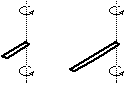## 20091109

### Physics quiz question: 1-m stick versus 2-m stick

Physics 205A Quiz 5, fall semester 2009
Cuesta College, San Luis Obispo, CA

Cf. Giambattista/Richardson/Richardson, Physics, 2/e, Problem 8.3A 1.0 m ruler and a 2.0 m ruler are made of the same material (and therefore the 2.0 m ruler is twice as massive). What is the ratio of the rotational inertia of the 1.0 m ruler to the 2.0 m ruler, if they are both pivoted to swing about their ends?
(A) 0.063.
(B) 0.13.
(C) 0.25.
(D) 0.50.

The rotational inertia of a uniform rod of mass M and length L pivoted about one end is given by:

I = (1/3)·M·L2.

With M2 = 2·M1, and L2 = 2·L1, then the ratio of I1 to I2 is then:

I1/I2 = ((1/3)·M1·L12)/((1/3)·M2·L22) = (M1/M2)·(L1/L2)2 = (1/2)·(1/2)2 = (1/2)3 = 0.125,

which is response (B), to two significant figures. Response (A) is (1/2)4 to two significant figures; response (C) is (1/2)2; response (D) is (1/2).

Student responses
Sections 70854, 70855
(A) : 0 students
(B) : 9 students
(C) : 27 students
(D) : 12 students

Success level: 18%
Discrimination index (Aubrecht & Aubrecht, 1983): 0.11

Student responses
Section 72177
(A) : 0 students
(B) : 5 students
(C) : 2 students
(D) : 5 students

Success level: 41%
Discrimination index (Aubrecht & Aubrecht, 1983): 0.80